Select Page

# MCQ in English Solutions for CBSE Maths 12 Science Relations and Functions

MCQ in English Solutions for CBSE Maths 12 Science Relations and Functions to enable students to get Solutions in a narrative video format for the specific question.

Expert Teacher provides MCQ Solutions for CBSE Maths 12 Science Relations and Functions through Video Solutions in English language. This video solution will be useful for students to understand how to write an answer in exam in order to score more marks. This teacher uses a narrative style for a question from Relations and Functions not only to explain the proper method of answering question, but deriving right answer too.

Please find the question below and view the Solution in a narrative video format.

Question:

Solution Video in English:

You can select video Solutions from other languages also. Please check Solutions in ( Hindi )

## Similar Questions from CBSE, 12th Science, Maths, Relations and Functions

Question 1 : Letdefined as f(x) = x be an identity function. Then, (View Answer Video)

Question 2 : A function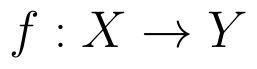is bijective if and only if, (View Answer Video)

Question 3 : Let * be the binary operation on N given by a * b = LCM of a and b. Find 20 * 16. (View Answer Video)

Question 4 : Functions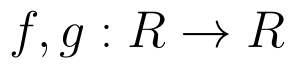are defined respectively, by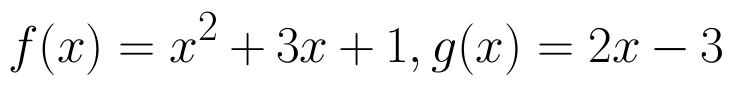, find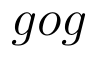(View Answer Video)

Question 5 : Let A = {1, 2, 3}. Then, number of relations containing (1, 2) and (1, 3) which are reflexive and symmetric but not transitive is, (View Answer Video)

### Continuity and Differentiability

Question 1 :  Find the second order derivative of the function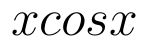. (View Answer Video)

Question 2 : If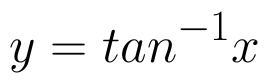, find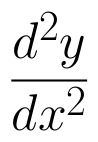in terms of y alone. (View Answer Video)

Question 3 : Differentiate the function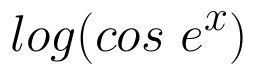with respect to x. (View Answer Video)

Question 4 : Differentiate w.r.t.x the function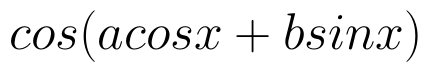, for some constant a and b. (View Answer Video)

Question 5 : Differentiate the function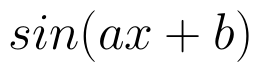with respect to x. (View Answer Video)

### Matrices

Question 1 : If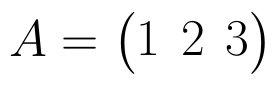, write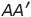, where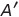is the transpose of A? (View Answer Video)

Question 2 : Using elementary transformation, find the inverse of the matrix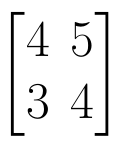. (View Answer Video)

Question 3 : Given,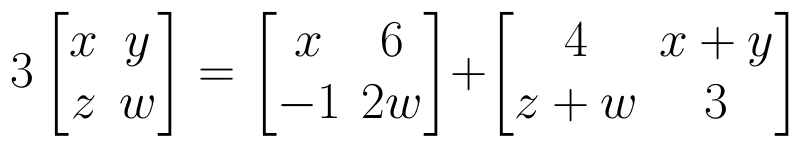, find the value of z. (View Answer Video)

Question 4 : Find the value of x,  from the equation: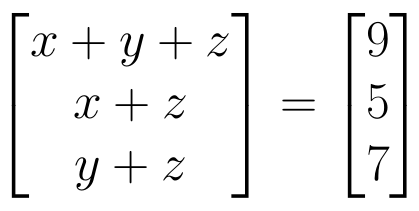. (View Answer Video)

Question 5 : Find the value of z,  from the equation: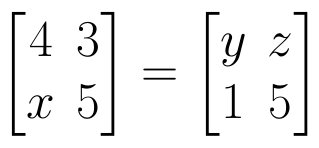(View Answer Video)

### Vector Algebra

Question 1 : Find a vector in the direction of vector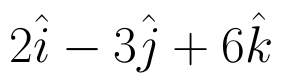which has magnitude 21 units.  (View Answer Video)

Question 2 : Find the projection of the vector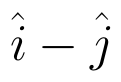on the vector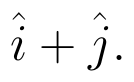(View Answer Video)

Question 3 : Find the scalar components of the vector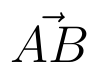with initial point A (2, 1) and terminal point B (-5, 7).  (View Answer Video)

Question 4 : Find a vector in the direction of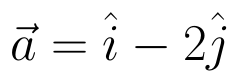that has magnitude 7 units.   (View Answer Video)

Question 5 : If the vertices A, B, C of a triangle ABC have position vectors (1, 2, 3), (-1, 0, 0), (0, 1, 2) respectively then find <ABC (<ABC is the angle between the vectors BA and BC). (View Answer Video)